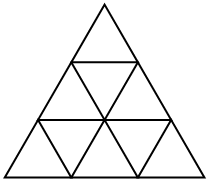# Counting parallelograms

The following problem appeared in the 1991 CMO, and it has a particularly clever solution.

In the figure, the side length of the large equilateral triangle is $3$ and $f(3)$, the number of parallelograms bounded by sides in the grid, is $15$. For the general analogous situation, find a formula for $f(n)$, the number of parallelograms, for a triangle of side length $n$.[Show Solution]